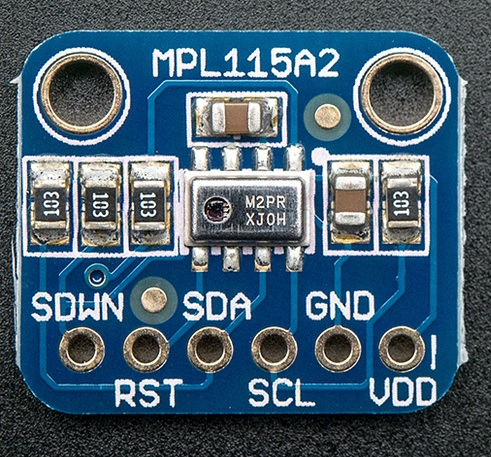# UPM

The UPM API is a high level sensor library for IoT devices using MRAA. See examples here. Back to index page.
SparkFun sensor images provided under CC BY-NC-SA-3.0.

# MPL3115A2 Class

Module: mpl3115a2

Freescale Semiconductor* MPL3115A2 is a high-precision, ultra-low power consumption pressure sensor. Its operating range is 50-110 kPa.## Methods

### `MPL3115A2`

(
• `bus`
• `devAddr`
• `mode`
)
Number

Instantiates an MPL3115A2 object

#### Parameters:

• `bus` Number

Number of the used bus

• `devAddr` Number

Address of the used I2C device

• `mode` Number

MPL3115A2 oversampling (6 = 64x)

Number:

### `testSensor`

() Number

MPL3115A2 object destructor; basically, it closes the I2C connection. ~MPL3115A2(); no need for this here, as the I2c connection will be closed when the m_i2ControlCtx variable will go out of scope Tests the sensor and tries to determine if the sensor is operating by looking for small variations in the value

Number:

### `resetSensor`

() Number

Performs a soft reset of the MPL3115A2 device to ensure it is in a known state. This function can be used to reset the min/max temperature and pressure values.

Number:

### `dumpSensor`

()

Dumps out the I2C register block to stdout

### `sampleData`

() Number

Initiates a temperature/pressure mesasurement and waits for the function to complete. Temperature and pressure registers can be read after this call.

Number:

### `getPressureReg`

(
• `reg`
)
Number

Reads the pressure value from MPL3115A2 [Pa * 100]

#### Parameters:

• `reg` Number

Base address of the pressure register

Number:

### `getTempReg`

(
• `reg`
)
Number

Reads the temperature value from MPL3115A2 [degC * 1000]

#### Parameters:

• `reg` Number

Base address of the temperature register

Number:

### `getPressure`

(
• `bSampleData`
)
Number

Reads the current pressure value from MPL3115A2 [Pa]

#### Parameters:

• `bSampleData` Number

Sets non-zero to a sample reading

Number:

### `getTemperature`

(
• `bSampleData`
)
Number

Reads the current temperature value from MPL3115A2 [degC]

#### Parameters:

• `bSampleData` Number

Sets non-zero to a sample reading

Number:

### `getSealevelPressure`

(
• `altitudeMeters`
)
Number

Reads the current pressure and, using a known altitude, calculates the sea level pressure value [Pa] This function should be preceded by the sampleData() call

#### Parameters:

• `altitudeMeters` Number

Altitude in meters

Number:

### `getAltitude`

(
• `sealevelPressure`
)
Number

Reads the current pressure and, using a known sea level pressure, calculates the altitude value [m] This function should be preceded by the sampleData() call

#### Parameters:

• `sealevelPressure` Number

Current sea level pressure

Number:

### `setOversampling`

(
• `oversampling`
)

Defines the oversampling setting (ranges from 0 to 7). The value represents 2^n samples (ranging from 1 to 128). The time to calculate a sample is approximately (2^n * 4 + 2) ms

#### Parameters:

• `oversampling` Number

New oversampling value

### `getOversampling`

() Number

Returns the current oversampling value

Number:

### `getTemperatureMax`

() Number

Reads the maximum measured temperature [degC]

Number:

### `getTemperatureMin`

() Number

Reads the minimum measured temperature [degC]

Number:

### `getPressureMax`

() Number

Reads the maximum measured pressure [Pa]

Number:

### `getPressureMin`

() Number

Reads the minimum measured pressure [Pa]

Number:

### `convertTempCtoF`

(
• `fTemp`
)
Number

Converts temperature from degC1000 to degF1000

#### Parameters:

• `fTemp` Number

Temperature in degC

Number:

### `convertPaToinHg`

(
• `fPressure`
)
Number

Converts pressure from Pa100 to inHg10000 This is set for 15degC (Pa = 0.0002961 in Hg) TODO: Change the function to add temperature calibration

#### Parameters:

• `fPressure` Number

Pressure in Pa

Number:

### `i2cWriteReg`

(
• `reg`
• `value`
)
Mraa::Result

Writes one byte to an I2C register

#### Parameters:

• `reg` Number

• `value` Number

Byte to be written

Mraa::Result:

### `i2cReadReg_16`

(
• `reg`
)
Number

Reads two bytes from an I2C register

#### Parameters:

• `reg` Number

Number:

### `i2cReadReg_8`

(
• `reg`
)
Number

• `reg` Number